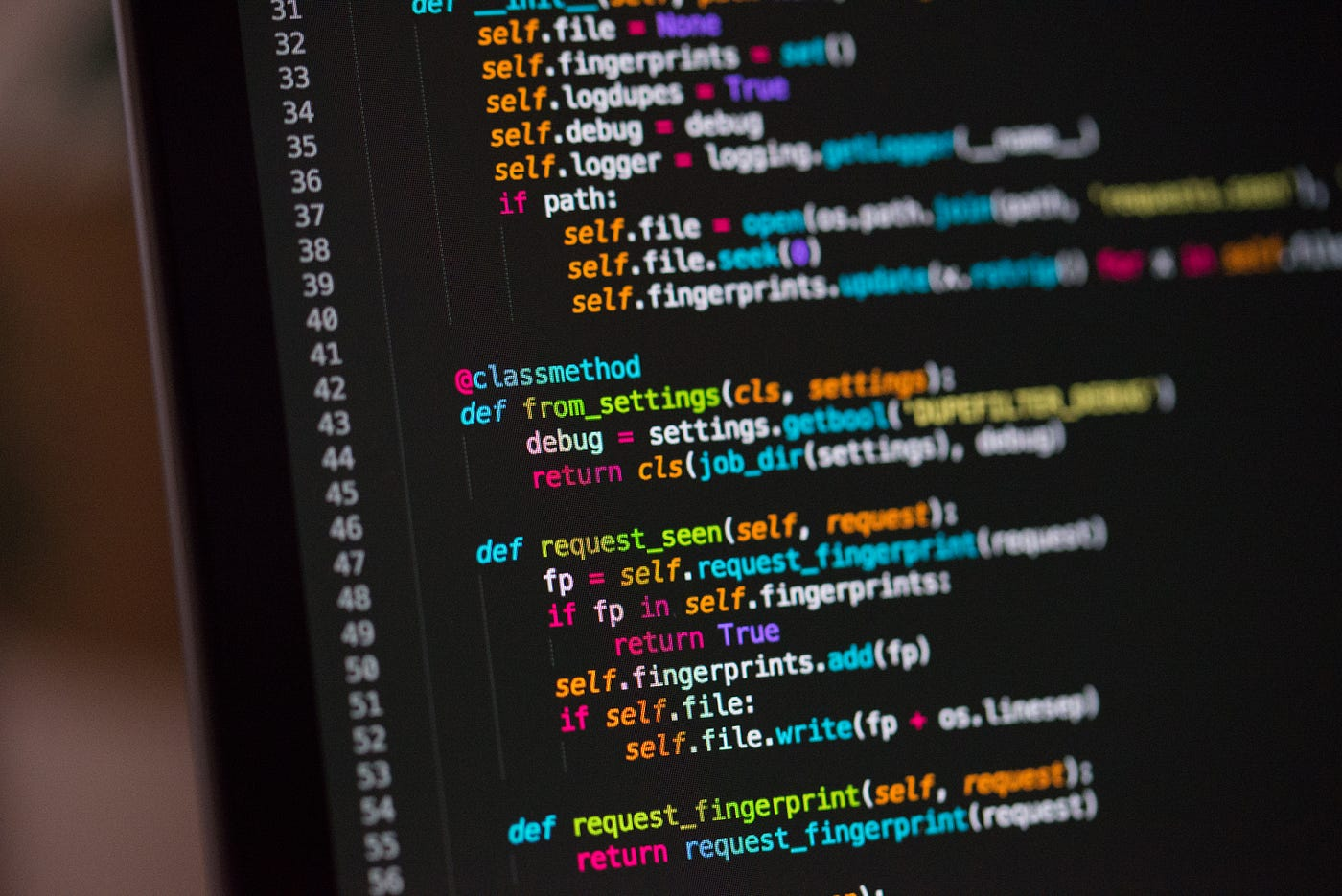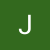# The Blind 75 Leetcode Series: Validate Binary Search TreePhoto by Chris Ried on Unsplash

Today, we are working on 98. Validate Binary Search Tree

Given the `root` of a binary tree, determine if it is a valid binary search tree (BST).

A valid BST is defined as follows:

The left subtree of a node contains only nodes with keys less than the node’s key.

The right subtree of a node contains only nodes with keys greater than the node’s key.

Both the left and right subtrees must also be binary search trees.

This is our first time dealing with binary search tree, or BST. BST is a unique tree that has several important characteristics, stated as above. To elaborate on these…

1. For a given node on BST, the nodes on the right branch, no matter how many levels deep, always have larger values than this given node
2. Similarly, all nodes on the left branch of the node always have smaller values.
3. The tree itself can be unbalanced (i.e. not all nodes at all levels, except the last one, have to be present. In other words, the tree doesn’t have to be a “complete tree”)
4. Because of the characteristics listed in 1 and 2, we can derive that all values should be unique. You should not see a duplicated value in 2 or more nodes. Otherwise it doesn’t qualify as a BST.
5. An empty tree (1 node with no value or no node at all) qualify as BST.

So to get more info from the interviewers, some questions to ask would be

1. Do we expect empty tree?
2. Do we need to treat missing branches in a special way? (ex. treat the tree as a complete tree and fill the missing nodes with some values)

If the interviewers tell me that we do expect empty tree and don’t worry about missing nodes (most likely the case), we can just start.

The question tests our understanding of BST. Can we transform the uniqueness of BST into our advantage?

All values are unique in a BST, and all values on the left side are smaller while values on the right side are larger. It makes sense if we try to read the nodes on the left first.

The order of reading should be:

1. read all nodes on the left side
2. read the node itself
3. read all nodes on the right side

If you’ve ever worked on tree traversals before, you might see that this is the in-order traversal method.

Take a look of the definitions of 3 different types of traversals with visual aid.

The easiest way to set up an in-order traversal is through recursion. For each iteration, we always check if the node has a left child until there’s none, then we record the number, and then we check for the right child.

`def inOrder(root, results):  # results: list, root: node    if not root:        return    if root.left:        inOrder(root.left, results)    results.append(root.val)    if root.right:        inOrder(root.right, results)`

When we have gone through the entire tree, we will have all the nodes’ values stored in `results`. Then what? If this tree is indeed a BST, the way we keep accessing the left children will result in smaller values to be stored before the larger ones, all in ascending order. Therefore, we just need to check if the values are sorted in ascending order. If the answer is yes, then we know this tree is indeed a valid BST. If not, it’s not valid.

To put everything into code:

`def inOrder(root, results):    if not root:        return    if root.left:        inOrder(root.left, results)    results.append(root.val)    if root.right:        inOrder(root.right, results)        def isValidBST(root):    results = []    if not root:        return results    inOrder(root, results)    # check if lower idx values < higher index value    for i in range(1, len(results)):        if results[i-1] >= results[i]:            return False    return True`

What’s the time complexity for this?

We traverse through the entire tree, and then we go through the `results` which is also the size of the tree. If the size of the tree is n, then our time complexity is O(n).

We are using recursion with a tracker, `results` , for our initial approach. Can we do it without?

Again, we have to think about the property. If we are just comparing the values, we can pass in the boundaries to each recursion instead of the list. We can do the check right at each recursion and return early if we identify that such tree is not BST.

We first use the positive and negative infinities as the boundaries. When we check the left child, we use the root value as the right boundary, and left_child.value should be less than right boundary and greater than left boundary. For the right child, we use root.value as the left boundary instead.

The base case here is when we no longer see a node. Then we have reached the leaf node. Return true from that point. As the results propagate up, we eventually get to the root node and we return the answers.

`def inOrder(root, left, right):    if not root:        return True    if left >= root.val or right <= root.val:        return False        return inOrder(root.left, left, root.val) and inOrder(root.right, root.val, right)        def isValidBST(root):    return inOrder(root, float('-inf'), float('inf'))`

Last but not least, whenever we can just a recursion to keep drilling down the tree, we should be able to transform it into an iterative approach using a while-loop.

`def isValidBST(root):    previous = None    stack = []    while 1:        # keep inserting left children to stack until leaf node        while root:            stack.append(root)            root = root.left        # pop the last-inserted (closer to leaf) node from stack. If can't, we've reached the end of stack, it's a valid BST        if not stack:            return True        node = stack.pop()        # since we've been going left, the val in previous should be smaller than the current node        if previous and previous.val >= node.val:            return False        # in first iteration, we go straight here since there's no previous, set the last-inserted node as previous, then we go to the right child        previous = node        root = node.right`

I personally find the first approach the most intuitive, but it’s completely up to you which to choose unless there’s a requirement to, for example, not to use recursion (which I find it stupid to ask).

Most of the time, interviewers won’t straight tell you to traverse through a BST. It’s packaged/decorated in a more complicated problem. Just make sure to look for BST’s characteristics to know if you’re dealing with a BST. Know which direction you are traversing and know the comparison boundaries. Make sure you don’t confuse ≤ with <.

That’s it! Another problem down.